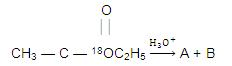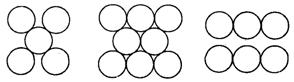Click to Chat

1800-1023-196

+91-120-4616500

CART 0

• 0

MY CART (5)

Use Coupon: CART20 and get 20% off on all online Study Material

ITEM
DETAILS
MRP
DISCOUNT
FINAL PRICE
Total Price: Rs.

There are no items in this cart.
Continue Shopping• Complete JEE Main/Advanced Course and Test Series
• OFFERED PRICE: Rs. 15,900
• View Details

```IIT-JEE-Chemistry-Mains–2000

MAINS

Time : Two hours                                                              Max. Marks : 100
_______________________________________________________________________

General Instructions :
1. There are ten questions in this paper. Attempt all Questions.
2. Answer each question starting on a new page. The corresponding question number must be written in the left margin. Answer all the parts of a question at one place only.
3. Use only Arabic numerals (0, 1, 2 ………….9) in answering the questions irrespective of the language in which your answer.
4. Use of logarithmic tables is not permitted.
5. Use of calculator is NOT PERMITTED.
_______________________________________________________________________

1. (a) Write the chemical reactions associated with the ‘brown ring test’.

(b) Draw the structures of [Co(NH3)6]3+, [Ni(CN)4]2– and [Ni(CO)4]. Write

thehybridization of atomic orbitals of the transition metal in each case.

(c) An aqueous blue coloured solution of a transition metal sulphate reacts with H2S in acidic medium to give a black precipitate A, which is insoluble in warm aqueous solution of KOH. The blue solution on treatment with KI in weakly acidic medium, turns yellow and produces a white precipitate B. Identify the transition metal ion. Write the chemical reactions involved in the formation of A and B.

2. (a) Give an example of oxidation of one halide by another halogen. Explain the feasibility of the reaction.

(b) Write the MO electron distribution of O2. Specify its bond order and magnetic property.

(c) 92238U is radioactive and it emits a and b particles to form 82206Pb. Calculate the number of a and b particles emitted in this conversion. An ore of 92238U is found to contain 92238U and 82206Pb in the weight ratio of 1 : 0.1. The half-life period of92238U is 4.5 × 109 years. Calculate the age of the ore.

3. (a) (i) Give an example of oxidation of one halide by another halogen. Explain the feasibility of the reaction.
(ii) Write the balanced chemical equation for developing photographic films.

(b) Write the chemical reactions associated with the ‘borax bead test’ of cobalt (II) oxide.

(c) Draw the molecular structures of XeF2, XeF4 and XeO2F2, indicating the location of lone pair(s) of electrons.

4. (a) Identify the pairs of enantiomers and diastereomers from the following compounds I, II and III.(b) Give reasons for the following :
(i) tert-Butylbenzene does not give benzoic acid on treatment with acidic KMnO4.
(ii) CH2 = CH– is more basic than HC º C–.
(iii) Normally, benzene gives electrophilic substitution reaction rather than electrophilic addition reaction although it has double bonds.

(c) What would be the major product in each of the following reactions?5. (a) Write the structures of the products A and B.(b) How would you bring about the following conversion (in 3 steps)?
Aniline ® Benzylamine

(c) An organic compound A, C6H10O, on reaction with CH3MgBr followed by acid treatment gives compound B. The compound B on ozonolysis gives compound C, which in presence of a base gives 1-actetyl cyclopentene D. The compound B on reaction with HBr gives compound E. Write the structures of A, B, C and E. Show how D is formed from C.

6. (a) Write the structures of alkaline at pH = 2 and pH = 10.

(b) Identify A, B and C, and give their structures.(c) An organic compound A, C8H4O3, in dry benzene in the presence of anhydrous AICI3 gives compound B. The compound B on treatment with PCI5, followed by reaction with H2/Pb(BaSO4) gives compound C, which on reaction with hydrazine gives a cyclised compound D (C14H10N2). Identify A, B, C and D. Explain the formation of D from C.

7. (a) The average concentration of SO2 in the atmosphere over a city on a certain day is 10 ppm, when the average temperature is 298 K. Given that the solubility of SO2 in water at 298 K is 1.3653 moles litre–1 and pKa of H2SO3 is 1.92, estimate the pH of rain on that day.

(b) Calculate the pressure exerted by one mole of CO2 gas at 273 K if the Van der Waals constant a = 3.592 dm6 atm mol–2. Assume that the volume occupied by CO2molecules is negligible.

(c) The figures given below show the location of atoms in three crystallographic planes in a FCC lattice. Draw the unit cell for the corresponding structures and identify these planes in your diagram.8. (a) A hydrogenation reaction is carried out at 500 K. If the same reaction is carried out in the presence of a catalyst at the same rate, the temperature required is 400 K. Calculate the activation energy of the reaction if the catalyst lowers the activation barrier by 20 kJ mol–1.

(b) Copper sulphate solution (250 mL) was electrolysed using a platinum anode and a copper cathode. A constant current of 2 mA was passed for 16 minutes. It was found that after electrolysis the absorbance of the solution was reduced to 50% of its original value. Calculate the concentration of copper sulphate in the solution to begin with.

(c) Calculate the energy required to excite one litre of hydrogen gas at 1 atm and 298 K to the first excited state of atomic hydrogen. The energy for the dissociation of H — H bond is 436 kJ mol–1.

9. (a) A sample of argon gas at 1 atm pressure and 27oC expands reversibly and adiabatically from 1.25 dm3 to 2.50 dm3. Calculate the enthalpy change in this process. CV, m for argon is 12.49 J K–1 mol–1.

(b) To 500cm4 of water, 3.0 × 10–3 kg of acetic acid is added. If 23% of acetic acid is dissociated, what will be the depression in freezing point? Kf and density of water are 1.86K kg–1 mol–1 and 0.997gcm–3, respectively.

(c) Show that the reaction CO(g) + (1/2)O2(g) --> CO2(g) at 300 K, is spontaneous and exothermic, when the standard entropy change is –0.094 kJ mol–1 K–1. The standard Gibbs free energies of formation for CO2 and CO are –394.4 and –137.2 kJ mol–1, respectively.

10. (a) Give reasons(s) why elemental nitrogen exists as a diatomic molecular whereas elemental phosphorus is a tetraatomic molecule.

(b) Give the structures of the products in each of the following reaction.(c) The following electrochemical cell has been set up.
Pt(1)|Fe3+, Fe2+ (a = 1)| Ce4+, Ce3+ (a = 1)|Pt(2)
EO(Fe3+, Fe2+) = 0.77 V and EO(Ce4+, Ce3+) = 1.61 V.

(d) Diborane is a potential rocket fuel which undergoes combustion according to the reaction
B2H6(g) + 3O2(g) --> B2O3(s) + 3H2O(g)

From the following data, calculate the enthalpy change for the combustion of diborane.
2B(s) + (3/2) O2(g) --> B2O3(s)                     DH = –1273 kJ mol–1
H2(g) + (1/2) O2(g) --> H2O(l)                       DH = –286 kJ mol–1
H2O(l) --> H2O(g)                                        DH = 44 kJ mol–1
2B(s) + 3H2(g) --> B2H6(g)                           DH = 36 kJ mol–1
```### Course Features

• 731 Video Lectures
• Revision Notes
• Previous Year Papers
• Mind Map
• Study Planner
• NCERT Solutions
• Discussion Forum
• Test paper with Video Solution